Exponentials

Exponentials

 Site: TBAISD Moodle Course: Michigan Algebra II Preview 2012 Book: Exponentials Printed by: Guest user Date: Friday, January 15, 2021, 10:42 PM

Method 1

There are two methods for solving exponential equations. The first method requires that the bases in both exponentials are the same. If the two sides of the original equation do not have the same base, it is sometimes possible to rewrite them with the same base. This method uses an exponential property which states:

If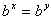then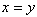.

Example 1 Solve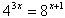Step 1. Rewrite each side with a common base.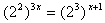Step 2. Use the Power of a Power Property.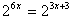Step 3. Since the bases are the same, equate the exponents.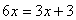Step 4. Solve for x.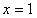Example 2

Solve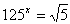Step 1. Rewrite each side with a common base.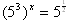Step 2. Use the Power of a Power Property.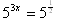Step 3. Because the bases are the same, equate the exponents.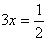Step 4. Solve for x.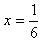Method 2

The second method for solving exponential equations involves using logarithms. For example, when trying to solve 3x = 11, 3 and 11 cannot be written as like bases. The log of both sides will need to be determined.

Example 1 Solve the equation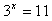Step 1. Since there is no common base, apply logarithms.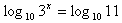In this example, common logs were used but any base can be used.

Step 2. Use the Power to a Power Property.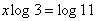Step 3. Solve for x.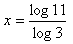*Note: If starting with a different base, the Change of Base Property will create this same equation.

Step 4. Use a calculator to find the answer.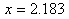Step 5. Make sure to check the answer: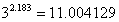*Note: Since the value of x was rounded to three decimal places it creates a round-off error (a slightly inaccurate answer when checked).

Example 2

Solve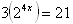Step 1. Isolate the exponential expression. First, divide both sides by 3.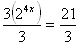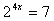Step 2. Since there is no common base, apply logarithms.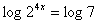Step 3. Use the Power to a Power Property.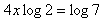Step 4. Solve for x.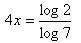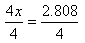Step 5. Use a calculator to find the answer.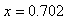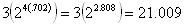Video Lesson

To learn how to solve exponential equations, select the following link:

Solving Exponential Equations

Sources

Sources used in this book:

Embracing Mathematics, Assessment & Technology in High Schools; a Michigan Mathematics & Science Partnership Grant Project

Holt, Rinehart, & Winston, "Solving Exponential Equations." http://my.hrw.com/math06_07/nsmedia/lesson_videos/alg2/player.html?contentSrc=6465/6465.xml (accessed 7/13/2010).